# A sample of plots

The idea of this notebook is to show off a number of plot types, and act as a simple check of the plotting output. It requires matplotlib and does not attempt to describe the plots (see the help for the plot constructor for this!).

:

import numpy as np

%matplotlib inline

:

from sherpa import data
from sherpa.astro import data as astrodata

from sherpa import plot
from sherpa.astro import plot as astroplot


## One dimensional data plots

:

x1 = [100, 200, 600, 1200]
y1 = [2000, 2100, 1400, 3050]

d1 = data.Data1D('oned', x1, y1)

plot1 = plot.DataPlot()
plot1.prepare(d1)
plot1.plot()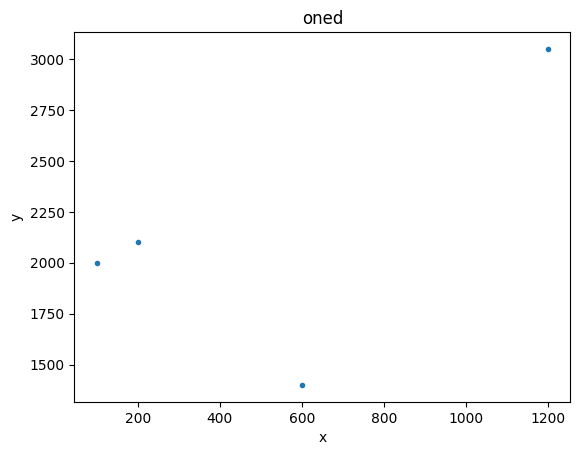We can have some fun with the plot options (these are a mixture of generic options, such as xlog, and ones specific to the plotting backend - which here is matplotlib - such as marker).

:

plot1.plot(xlog=True, linestyle='dotted', marker='*', markerfacecolor='orange', markersize=20, color='black')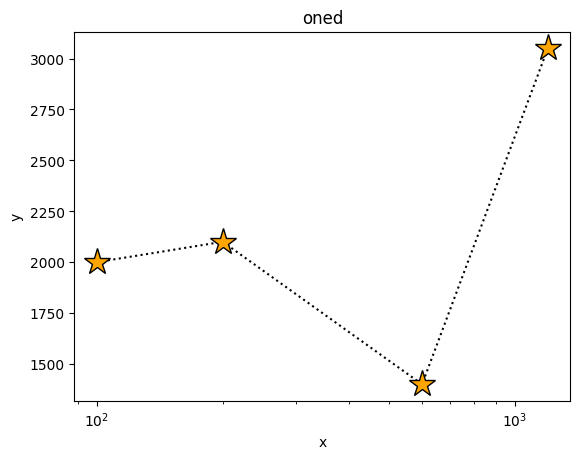The plot object contains the preferences - here we look at the default plot settings. Note that some plot types have different - and even multiple - preference settings.

:

plot.DataPlot.plot_prefs

:

{'xerrorbars': False,
'yerrorbars': True,
'ecolor': None,
'capsize': None,
'barsabove': False,
'xlog': False,
'ylog': False,
'linestyle': 'None',
'drawstyle': 'default',
'color': None,
'marker': '.',
'markerfacecolor': None,
'markersize': None,
'alpha': None,
'xaxis': False,
'ratioline': False,
'linecolor': None}


Error bars - here on the dependent axis - can be displayed too:

:

dy1 = [100, 50, 200, 300]
d2 = data.Data1D('errors', x1, y1, dy1)

plot2 = plot.DataPlot()
plot2.prepare(d2)

plot2.plot()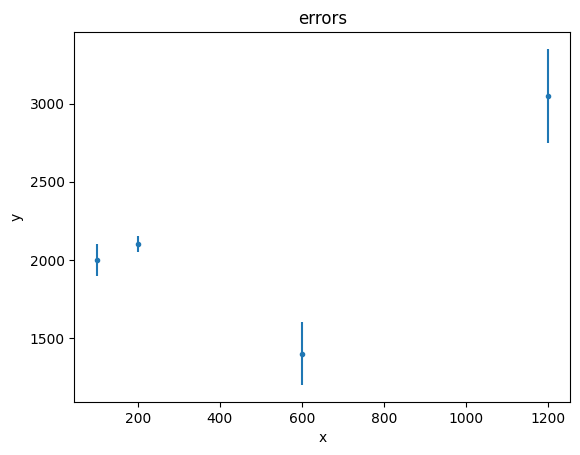:

plot2.plot(capsize=4)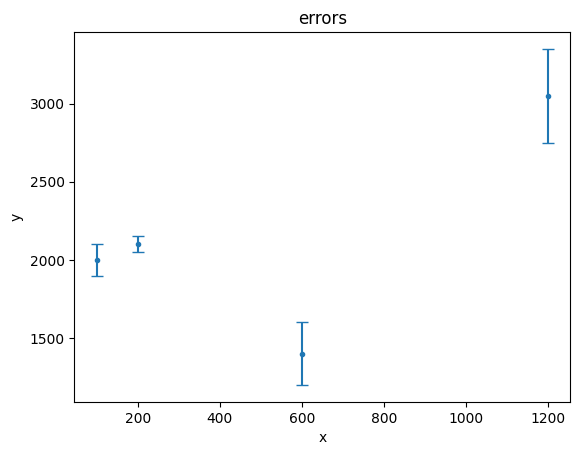Histogram-style data (with low and high edges) are handled similarly:

:

xlo2 = [0.1, 0.2, 0.4, 0.8, 1.5]
xhi2 = [0.2, 0.4, 0.6, 1.1, 2.0]
y2 = [10, 12, 3, 0, 4]

data3 = data.Data1DInt('int1', xlo2, xhi2, y2)

plot3 = plot.DataHistogramPlot()
plot3.prepare(data3)
plot3.plot(xlog=True)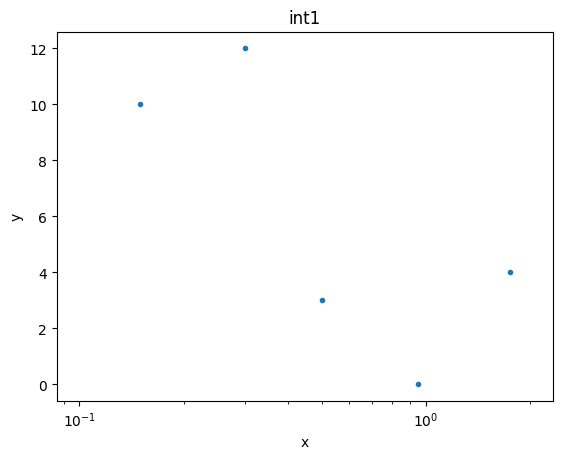If we want to see the data drawn “like a histogram” then we need to set the linestyle attribute:

:

plot3.plot(xlog=True, linestyle='solid')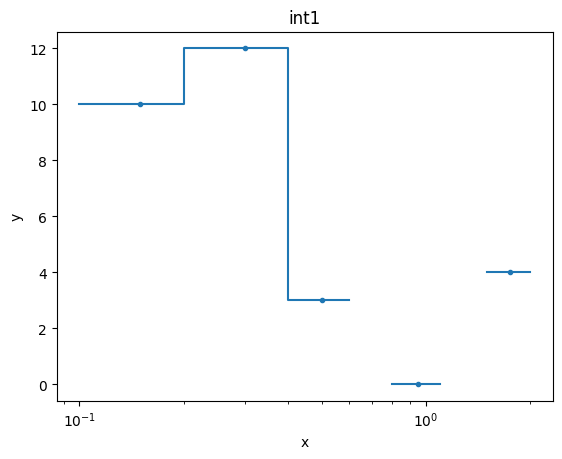The histogram-style plots are an example of a plot using a different name for the preference settings, in this case histo_prefs:

:

plot.DataHistogramPlot.histo_prefs

:

{'xerrorbars': False,
'yerrorbars': True,
'ecolor': None,
'capsize': None,
'barsabove': False,
'xlog': False,
'ylog': False,
'linestyle': 'None',
'drawstyle': 'default',
'color': None,
'alpha': None,
'marker': '.',
'markerfacecolor': None,
'markersize': None,
'linecolor': None}


Previously we explicitly set the error values, but we can also use one of the chi-square statistics to come up with error values. In this case it’s just the square-root of the data value (so, for $$x \sim 1$$ bin, we have an error of 0):

:

from sherpa.stats import Chi2DataVar

plot4 = plot.DataHistogramPlot()
plot4.prepare(data3, stat=Chi2DataVar())
plot4.plot(linestyle='dashed', marker=None, ecolor='orange', capsize=4)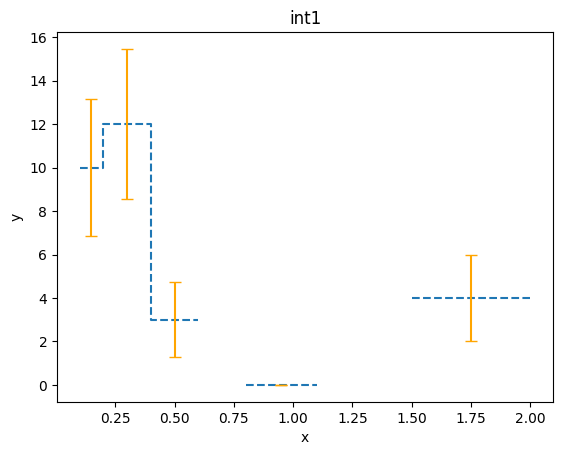## Object-less plots

There are a number of plot classes that don’t need a data object, such as scatter plots:

:

np.random.seed(1273)

# I've never used the Wald distribution before, so let's see how it looks...
#
z1 = np.random.wald(1000, 20, size=1000)
z2 = np.random.wald(1000, 2000, size=1000)

splot = plot.ScatterPlot()
splot.prepare(z1, z2, xlabel='z$_1$', ylabel='z$_2$', name='(z$_1$, z$_2$)')
splot.plot(xlog=True)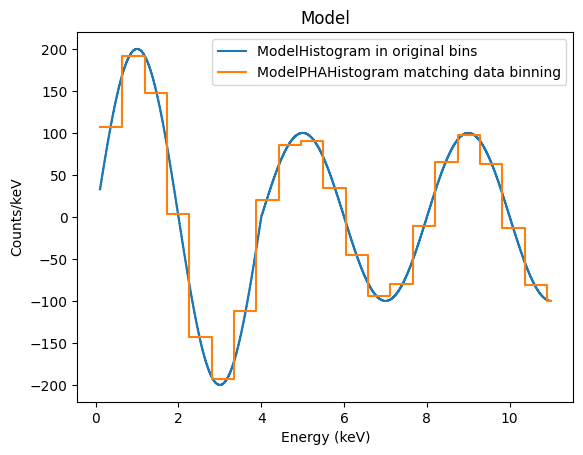and cumulative plots:

:

cplot = plot.CDFPlot()

cplot.prepare(z1, xlabel='z', name='z')
cplot.plot(xlog=True)

cplot.prepare(z2)
cplot.overplot()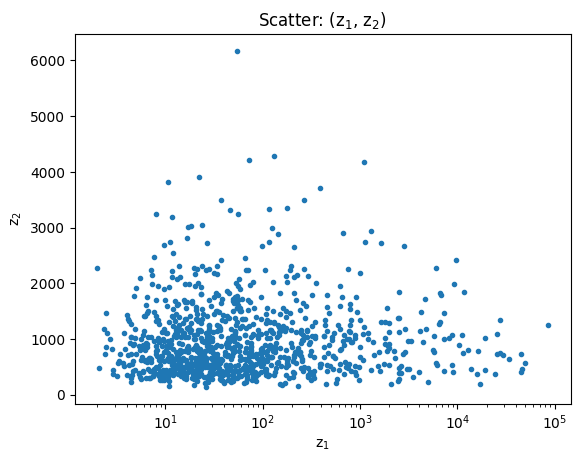Note that this is a small sampling of the available plot types (although most are variants of plotting either the data or a model).